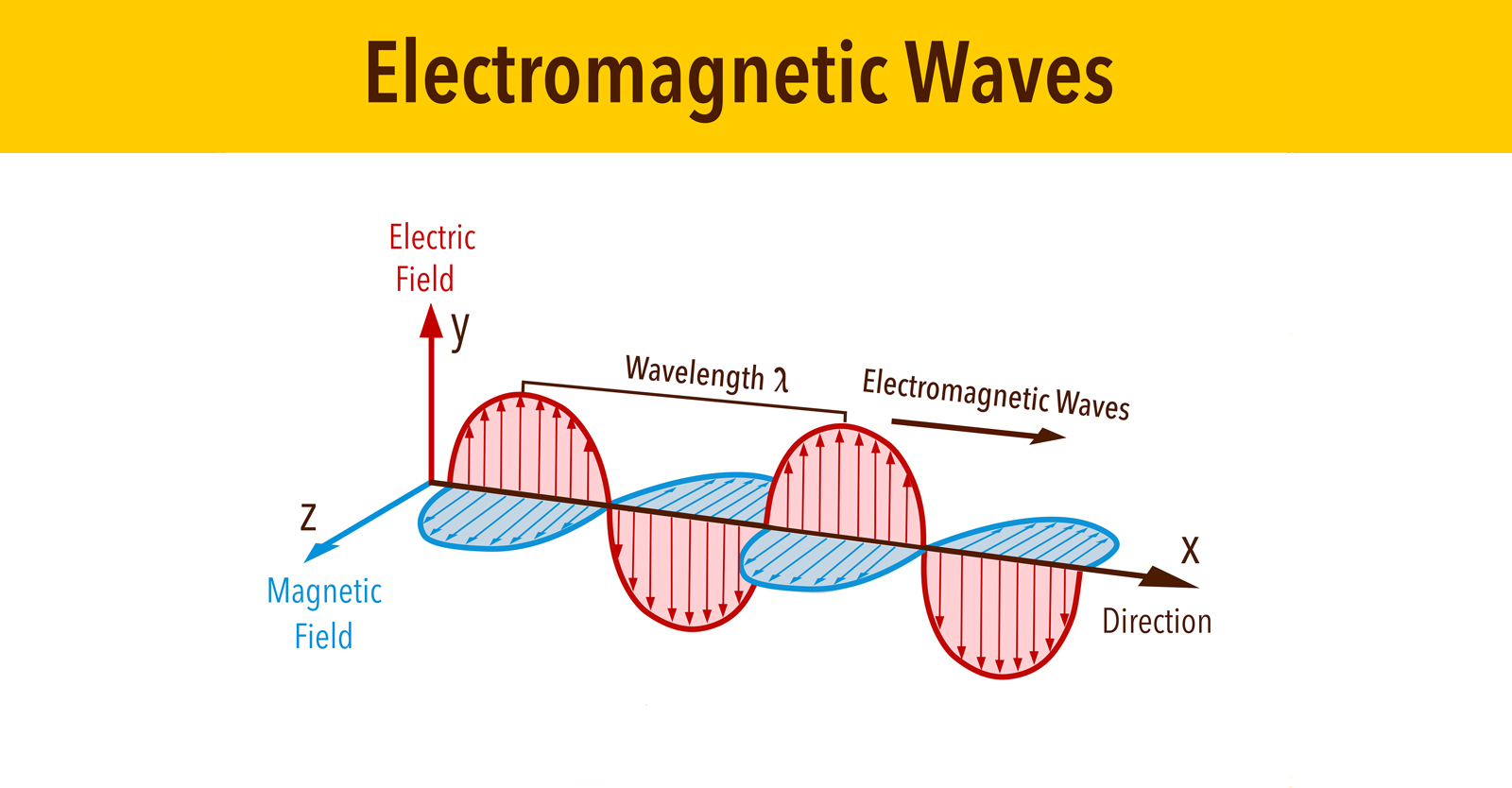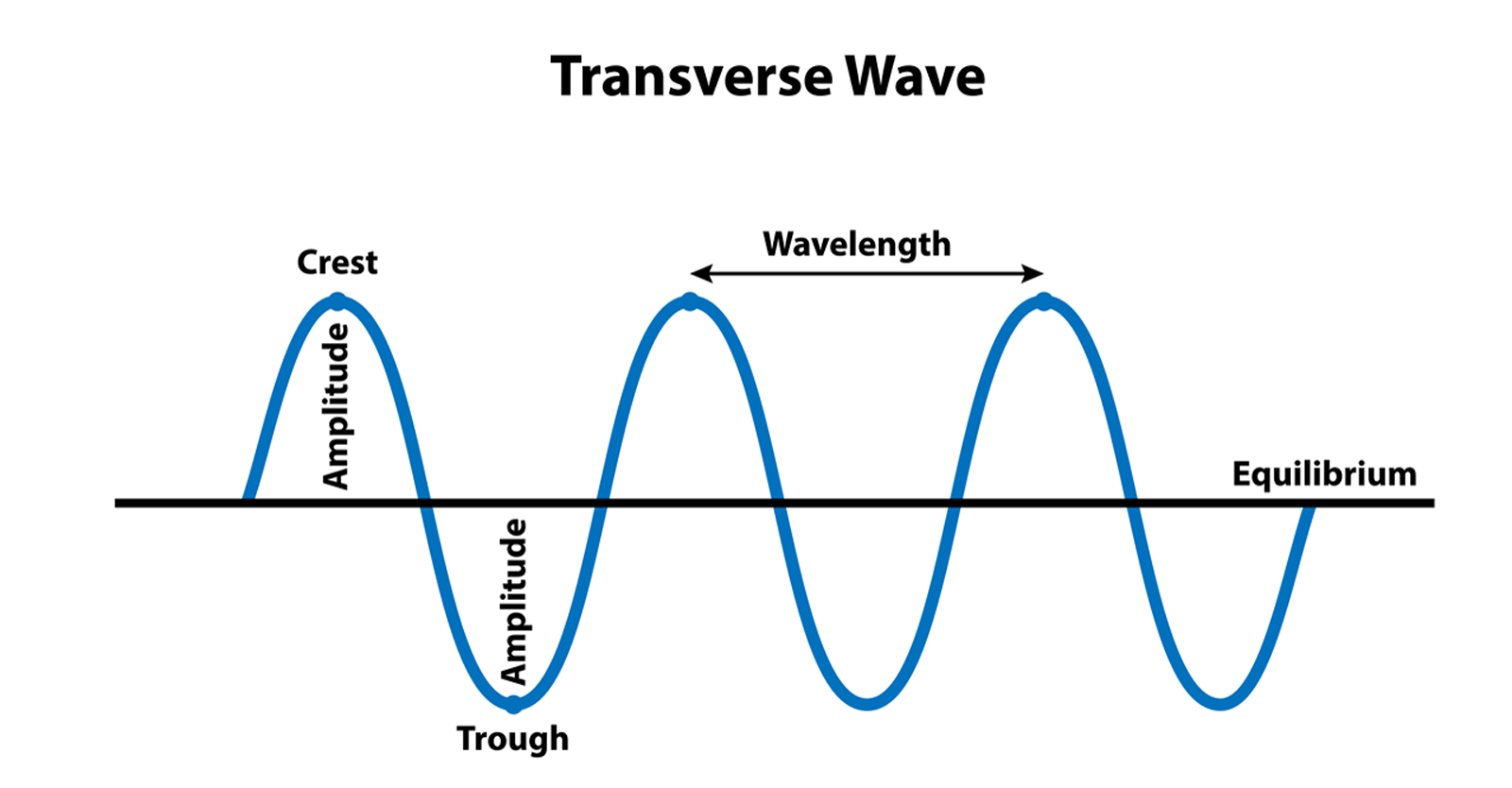# Physics

## Electromagnetism and Electromagnetic Force

Electromagnetism is the study of electromagnetic force, a fundamental force of nature that acts between charged particles. Charged particles interact in such a way that opposite charges attract, while like charges repel. At the most basic level, electromagnetism is the study of this fundamental interaction — the interaction between positively and negatively charged particles.

The electromagnetic force F that acts on a given charge q moving with a velocity ν in an electric field E and magnetic field B is given by the following equation:

#### F = q (E + ν × B)

Along with the electromagnetic force, the other fundamental forces of nature include the strong nuclear force, which describes the forces between protons within the atomic nucleus, the weak nuclear force, which governs particle decay processes such as radioactive decay, and the force of gravity, which is the study of the interaction between objects with mass.

## Electromagnetic Fields

In general, a “field” is any physical quantity that has different values at different points in space. Therefore, electromagnetic fields can be simply thought of as a mathematical function that describes the strength of the field and the direction of the field at different positions and times.

Electromagnetic fields make up electromagnetic radiation, such as light, and are also generated by charged particles, such as electrons. Electric fields are generated by a) stationary charges and b) variable or changing magnetic fields. Electric fields are measured in units of volts per meter (V/m). Magnetic fields are generated by a) moving charges and b) the intrinsic spin of elementary particles such as electrons. Magnetic fields are measured in units of Tesla (T) or Gauss (G). Electricity and magnetism are closely related components of the electromagnetic force, and therefore the study of electric and magnetic fields is fundamentally the study of the same subject. The intensity of electric and magnetic fields decreases with distance from the field source.

## Static Electromagnetic Fields

Static electromagnetic fields are electromagnetic fields that do not change in field strength or direction over time. They do not emit radiation and as such, are described as having a frequency of 0 Hertz (Hz).

Static electric fields, or electrostatic fields, are generated by stationary charges — charges that are not moving and fixed in space. Static electricity is an everyday phenomenon that occurs when charged particles are transferred between bodies. Since materials differ in their ability to transfer charge, some materials accumulate charge while others lose charge. When charged objects make contact with a neutrally-charged object, the negatively-charged object neutralizes its charge by donating electrons, while the positively-charged object neutralizes its charge by adopting electrons. This discharge may result in an electric discharge that can be felt or seen.

A familiar example of this occurs when walking across a carpet with socks on and making contact with a neutral object, such as the metal screw on a light switch. Static electric fields also describe the phenomenon of lightning. In a rain cloud, colliding and bumping water molecules create a static electric charge. When a significant build-up of charge occurs, it rapidly discharges via the neutral ground, resulting in lightning.

Another static electric field source is high-voltage direct current (DC) powerlines. A DC is an electric current whose flow of charge is always in the same direction. DC currents are also used in electronic devices that are powered via a battery or rechargeable devices like smartphones and laptops that use an alternating current (AC) adapter to convert from AC to DC.

Static magnetic fields or magnetostatic fields, are generated by magnets and charges flowing at a constant rate, such as DC devices. In magnetic materials such as iron, cobalt and nickel, the electrons produce a net magnetic field, whereas in non-magnetic materials, the magnetic fields generated by the electrons mostly cancel out resulting in extremely weak magnetic fields. In a magnet, the magnetic field extends from one end, the north pole, to the other end, the south pole. Similar to charged particles, like poles repel, while opposite poles attract. Static magnetic fields are also used for magnetic resonance imaging (MRI) and nuclear magnetic resonance (NMR).

## Variable Electromagnetic Fields

Variable electromagnetic fields are electromagnetic fields that change in magnitude or direction over time. As such, variable electric fields are defined as electric fields that change in magnitude or direction over time and are produced by variable magnetic fields.

Similarly, variable magnetic fields are defined as magnetic fields that change in magnitude or direction over time and are produced by variable electric fields. The magnetic field generated by the Earth at Earth’s surface is an example of a variable magnetic field, although it is commonly described as a static field because it changes at relatively large timescales. Over the last five million years, the Earth’s magnetic field has undergone up to 10 complete direction reversals. Additionally, variable magnetic fields can induce electric currents in conductors and in the human body. Common examples of variable magnetic field sources include AC devices that use electric currents that continuously change magnitude and periodically reverse direction. Examples of AC current devices include everyday electrical devices such as refrigerators, dishwashers and toasters as well as other infrastructure such as cell tower antennas.

Electromagnetic radiation (EMR) consists of oscillating electric and magnetic fields that propagate through space, as shown in the diagram below. Oscillation or oscillatory motion is defined as the periodic variation of magnitude or position around a central value, typically with respect to time. In the case of electromagnetic waves, oscillation refers to the variation of the electric and magnetic fields.## Electromagnetic Waves

The oscillations of a transverse wave are perpendicular (at right angles) to the direction of the propagation of the wave. As shown in the diagram above, the electric field is oscillating in the vertical direction, the magnetic field is oscillating in the horizontal direction and the wave is propagating in the x-direction.

The diagram below shows some of the key characteristics of transverse waves. The crest is the highest point on the wave, while the trough is the lowest point. The wave oscillates from equilibrium to the crest, back to the equilibrium, to the trough and back to the equilibrium, and continually repeats this oscillatory motion. The amplitude is given by the distance between the equilibrium and the crest, and the equilibrium and the trough.The wavelength of a transverse wave is the length of one complete wave cycle and is given by the distance between two adjacent and identical points on the wave. As shown in the diagram above, the wavelength can be determined by measuring the distance between two adjacent crests (or troughs). The wavelength is an important characteristic of electromagnetic radiation because it is closely related to the frequency and the energy of the radiation. Specifically, the wavelength and frequency are inversely proportional, meaning the longer the wavelength, the lower the frequency and the shorter the wavelength, the higher the frequency. The relationship between the wavelength (), frequency (f) and speed (c) of electromagnetic radiation is:

#### f λ = c

• The frequency (f) is measured in units of cycles per second or Hertz (Hz).
• The wavelength (λ) is measured in units of meters (m).
• The speed (c) is measured in units of meters per second or (m/s).

The electromagnetic radiation spectrum covers the entire range of electromagnetic radiation from radio waves, microwaves, infrared radiation, visible light, ultraviolet radiation, x-rays to gamma rays. In a vacuum, electromagnetic radiation travels at a constant speed, known as the speed of light. The frequency of electromagnetic radiation is proportional to the energy. In other words, as the frequency increases, the energy increases and as the frequency decreases, the energy decreases. The relationship between frequency (f) and energy (E) is given by:

#### E = hf

• The energy (E) is measured in units of joules (J).
• The frequency (f) is measured in units of cycles per second or Hertz (Hz).
• h is a constant known as Planck’s constant.

## Photon Model of Electromagnetic Radiation

So far, we have described electromagnetic radiation as oscillating electric and magnetic fields, or waves. Another model used to describe electromagnetic radiation is that of particles, called photons. A photon is a discrete packet (or quantum) of energy. The photon model of electromagnetic radiation has many useful applications in modern physics, and is also helpful when differentiating between ionizing and non-ionizing radiation. It is important to distinguish between ionizing and non-ionizing radiation because the type of radiation distinguishes between the types of damage that can occur within the body.

The ionizing radiation spectrum has higher frequencies and energies than non-ionizing radiation and is defined as radiation that has sufficient energy to remove electrons from atoms or molecules within matter. Alternatively, the non-ionizing radiation spectrum has lower frequencies and energies than ionizing radiation and does not have sufficient energy to remove electrons from atoms or molecules within matter. Ionization energy is defined as the amount of energy that is required to remove an electron from an atom or molecule.

## Ionization Energy and the Tetherball Analogy

The concept of ionization energy and the photon model of electromagnetic radiation can be better understood using an analogy of a tetherball. A tetherball is a playground game that consists of a ball suspended from the top of a vertical pole with a string, and tossed back and forth between players.

In this analogy, the atom or molecule is represented by the vertical pole, the electron is represented by the ball, the ionization energy is represented by the string and the photons are represented by the players. If the players, say wrestlers, start playing a game of tetherball, and upon tossing the ball to each other use so much force that the string breaks, this is analogous to ionizing radiation which has enough energy to break the bond between the electron and atom.

On the other hand, if the players, say children on a playground, toss the ball back and forth between themselves, and the string stays intact, this is analogous to non-ionizing radiation which does not have enough energy to break the bond between the electron and the atom. While this analogy does not describe all of the different types of damage that can occur from non-ionizing radiation, it illustrates a simple explanation of ionization energy.

Returning to the photon model of electromagnetic radiation, if individual photons have energies that are greater than the ionization energy of an atom or molecule, the photons can remove electrons, providing a direct avenue to cause damage to the atom or molecule. Alternatively, if individual photons have energies that are less than the ionization energy of the atom or molecule, the photons cannot remove electrons from the atom or molecule. However, while ionizing an atom or molecule is one way to cause damage in the body, many types of damage can occur at energies well below that of ionizing radiation.

Sign up for free news and updates from Robert F. Kennedy, Jr. and the Children’s Health Defense. CHD is implementing many strategies, including legal, in an effort to defend the health of our children and obtain justice for those already injured. Your support is essential to CHD’s successful mission.